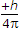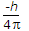# Electronics and Communication Engineering - Materials and Components

### Exercise :: Materials and Components - Section 4

26.

If there are no permanent dipoles in a dielectric material, the dielectric constant is independent of temperature.

 A. True B. False

Explanation:

No answer description available for this question. Let us discuss.

27.

Assertion (A): Magnetic susceptibility of copper is -0.5 x 10-5.

Reason (R): Copper is diamagnetic material.

 A. Both A and R are true and R is correct explanation of A B. Both A and R are true but R is not correct explanation of A C. A is true but R is false D. A is false but R is true

Explanation:

If magnetic susceptibility is very low μ, is nearly equal to 1 and the material is diamagnetic.

28.

An electron shell corresponding to principal quantum number n can contain a maximum of

 A. n2 electrons B. 2n2 electrons C. 2n electron D. n3 electron

Explanation:

The electron shell corresponding to the principal quantum number can contain no more than 2n2 electrons.

29.

A sheet of bakelite is inserted between the plates of an air capacitor. The capacitance will

 A. increase B. decrease C. remain the same D. may increase or decrease

Explanation:

Bakelite has higher permittivity than air.

30.

The spin angular momentum along a given direction is eitheror.

 A. True B. False